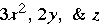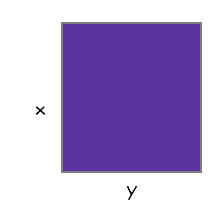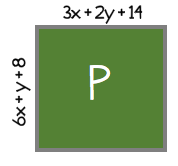# Simplifying Expressions

Now that we have learned how to translate verbal expressions into algebraic expressions, we will extend our knowledge of combining numbers and variables to simplifying these expressions. We have learned that a term is any product, quotient, or power of numbers and variables. Algebraic expressions that contain one or more terms are called polynomials.

### Examples

Polynomials with one term:  2xxy4x²

Polynomials with two terms:  x + 65a – bxyz +4

Polynomials with three terms:  x + y + zx + y – z

You can simplify polynomials by using grouping and combining like terms. If the terms have exactly the same variables to like powers, you can combine them to simplify the expressions.

Look at the following expression:

x = 1x

x + x = 2x

x + x + x = 3x

The numbers 1, 2, and 3 are coefficients of x.

An algebraic expression has terms that are separated by plus and minus signs. In the expression 3x + 6y, the terms are 3x and 6y. Check out the table below for some examples of terms.

Expression Terms
6x + 3y 6x and 3y
x – 2y x and 2y3a + 2b 3a and 2b

The terms of an expression can be combined to simplify that expression. Remember, only like terms can be combined.

6x and x (can combine because these are like terms)
Add or subtract the coefficients of the variable: 6x + 1x = 7x

3x – 3y – 2 (cannot combine any of these terms because they are different variables)

8x – 3x + 2 (can combine the 8x and the 3x – cannot combine the 2 with these variables)
Add or subtract the coefficients of the variable: 8x – 3x = 5x
Can rewrite the original expression: 5x + 2

*Always use the sign in front of the term to signify the operation.

Look at how the like terms are combined in the diagram.

8 + 3x + 2 + 4x + x + 4## Practice

Combine the like terms in each expression to rewrite the expression

1. 2x + 5 + 5x – 4 – x
2. 5 + 7x + 4x – 3 + 6
3. x + y + 2x – 3x + 2y + 3y
4. 3x² + 5x – 10 + 6x + 20

Now that you know how to combine like terms to simplify expressions, we are going to pick up the pace and learn to apply this skill to more complex expressions and add the distributive property to help find the equivalent expression. Are you ready?

### InvestigateCould we write an expression for the perimeter of the figure below? (Combine like terms in the expression). Could you use the distributive property to help? (yes)

To find the perimeter of a rectangle, you simply add all of the sides together. In this case, we have x + y + x + y and when we combine those terms, we get 2x + 2y. The formula for finding the perimeter of a rectangle is 2L + 2W. We could use the distributive property to combine our lengths and widths.Let us try the same thing with a triangle? Remember, just add all of the sides together as if you were going to build a fence around this triangle.

First, we need to combine all of the sides together. Our expression will look like this:

3x + 9 + x – 4 + 2x + 3.

Now, we can regroup our information so that the like terms are together.

(3x + x + 2x) + (9 – 4 + 3)

Finally, we simplify our terms.

6x + 8

The perimeter for this triangle is 6x + 8.

Which properties did you use to help you simplify the expression above?

One more shape and you will be ready to move to the next step in simplifying expressions! Use the numbers properties to find the perimeter of the figure below.In this rectangle, we can use the formula for Perimeter of a rectangle to simplify. (2L + 2W)

So, our expression would look like this:

2(6x + y + 8) + 2(3x + 2y + 14)

First, distribute all terms inside the parenthesis by the number outside the parenthesis:

2(6x) + 2(y) + 2(8) + 2(3x) + 2(2y) + 2(14)

Next, multiply the 2 by each term and rewrite without the parenthesis:

12x + 2y + 16 + 6x + 4y + 28

Now, use the commutative and associative properties to arrange your numbers for easier combinations:

(12x + 6x ) + (2y + 4y) + (16 + 28)

Finally, simplify each group of terms to find the perimeter of this rectangle.

18x + 6y + 44

## Practice

Combine the like terms in each expression to rewrite the expression

5.  2x² + 10x + 10 – 4x – x² + 1
6. 14y + 14x + 14 – 6x – 14
7. Find the perimeter of a rectangle with two sides of 4x and two sides of 8y.
8. Find the perimeter of a triangle with sides: 4x + 8, x – 3, 3x + 4
9. Find the perimeter of a polygon with sides: 5x² , 2x , 4x² , 6x
10. Find the perimeter of a rectangle with sides: 5x + 2y – 7 and 2x + 3y + 6

(source)